# Author: Ashish Mathur

There are 100 machines in a factory.  Every machine has different test frequency. In a given month, not every machine is tested but we still have the last known rating (from some previous month) of that machine.  We have to show the latest rating of each machine for each month in a stacked column chart. This way, the total number will remain 100 every month in the chart, but the rating distribution (color based on legend) will change based on last available rating of that machine.

For example, in January, 35 machines were tested. So we have latest ratings of these 35 machines. But as the rest of the machines also have some previous rating, the graph needs to show all 100, with last available rating.

The expected result should look like thisYou may download my PBI Desktop file from here.  The very same DAX formulas can be written in the DAX formula language of MS Excel as well.

Here is a simple 5 column dataset showing basic employee information.The objective is to show only those rows of data in which the Score (column D) is greater then 3.  While one can solve this with a simple filter, the solution will not be dynamic.  To get a dynamic solution, one may use the FILTER() dynamic array function that comes with the Microsoft 365 subscription service.

In cell G2, one may simply write this formula

=FILTER(A2:E9,D2:D9>3)

This is a far better solution because it is formula driven and thereby dynamic.  So all good till here.  Now let's make it a little interesting.

The revised objective is to show only those rows of data in which the Score (column D) is greater then 3 and only display 3 columns - Name, Department and Score (columns 1,3 and 4) in the end result.  This can be solved using Data > Advanced filter but the result will be static.  To get a dynamic solution, one may use a nested FILTER() function in cell G2

=FILTER(FILTER(A2:E9,D2:D9>3),{1,0,1,1,0})

This formula will return the same number of rows (3 rows) as the previous FILTER() function returned with only 3 columns - Name, Department and Score.  The 1's and 0's in the formula denote whether one would like to see the particular column in the end result or not.  So once again, all good till here.

Now, let's take it a notch higher.  What is there were 35 columns in the original dataset and one wanted to see data appearing in columns 1,6,22,25,29 and 34.  It will be quite time consuming to enter the 1's and 0's in order within the FILTER() function.  So the question here is how does one save time and effort?  Ideally one should be able to just enter the column headings one wants to see in the end result.As you can observe in the image above, one has to simply specify the columns to extract in column C and the result populates from column K rightwards and downwards.  Using dynamic array formulas and the FILTER() function, one saves effort in entering 1's and 0's in the FILTER() function (the FILTER() function has been written in cell K2 - download link of the file is shared below).  If one types Address in cell I5, then Address would automatically appear in cell N1 and so will the entries in range N2:N4.  So this does seem like a good solution.  So while it is good, it is not a perfect solution.  In column I, if one changes the order of the headings i.e. one enters Name, Dept, Address and Score (rather than Name, Dept, Score and Address), the result under columns M and N would be incorrect (see image below).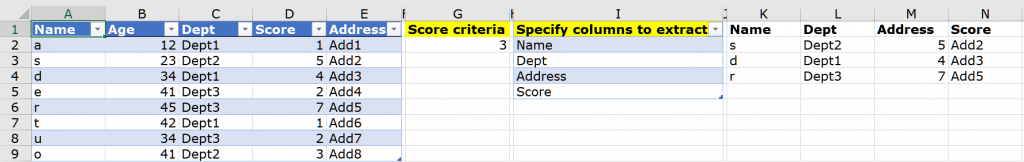Solving this challenge led me to using Data > Get & Transform.  I made use of the latest feature introduced in Power Query called "Data Types" (I received this feature update on December 4, 2020).As one can see in the image above, one simply has to select any heading one wants and that appears in the next available column.

You may download my solution workbook from here in which i have shown both formula based and the Power Query solution.

In this simple 3 column dataset, there are 2 accounts - Konto 1 and Konto 2.  Each account has a balance outstanding as on a certain date.  However, if you notice carefully, there is no balance for any account on January 4-5,9-10 2020.The objective is show the balance outstanding every day.  For days which are absent from the dataset, the balance outstanding should be the balance as on the previous day.  So for the Konto 1 account, on January 4-5, the balance should be 400 and on January 9-10, it should be 250.  The same logic applies for the Konto 2 account as well.  The expected result should beI have solved this problem using Data > Get & Transform and PowerPivot.  You may download my solution workbook from here.

Here's another related question.  Given this 3 column dataset, the objective is to determine the total of the "PDV Combined Total" column for the past "5 billing days" from today - the past 5 working days have to be determined by looking at the "IsABillingDay" column.  So if today is December 4, 2020, then the past 5 business days would be November 30, 2020 to December 4, 2020.  The result should be 14,95,937.  However, if today was December 2, 2020, then the past 5 business days would be November 24, 2020 to December 2, 2020.  The result would be 14,33,545.  You may download the solution in a PBI file from here.  So while this question has been solved using the DAX formula language in PowerBI Desktop, since the same formula language exists in MS Excel as well, this result can be obtained in MS Excel as well.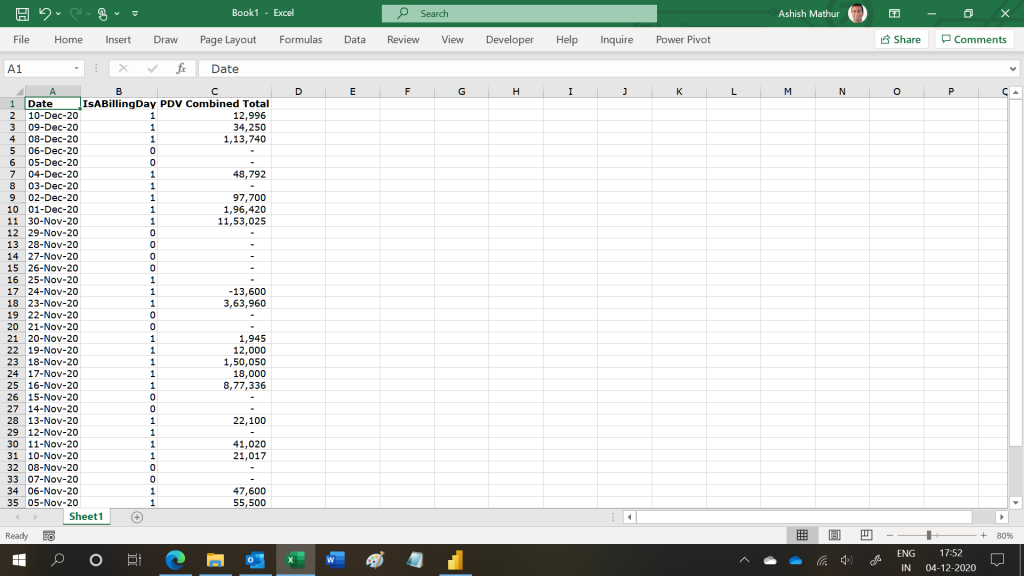Assume a simple 4 column dataset as shown below.  This data shows which ID had which type of subscription in which year.  So ID A, which started as a "Free" subscriber in 2018 switched to a "Premium" subscriber in 2019 and then churned out in 2020.  Likewise, ID D which started as a "Pro" subscriber in 2018, churned out in 2019 but returned as a "Free" subscriber in 2020.The objective is to study how subscribers switched from one subscription type to another across year.  So the expected result should look like this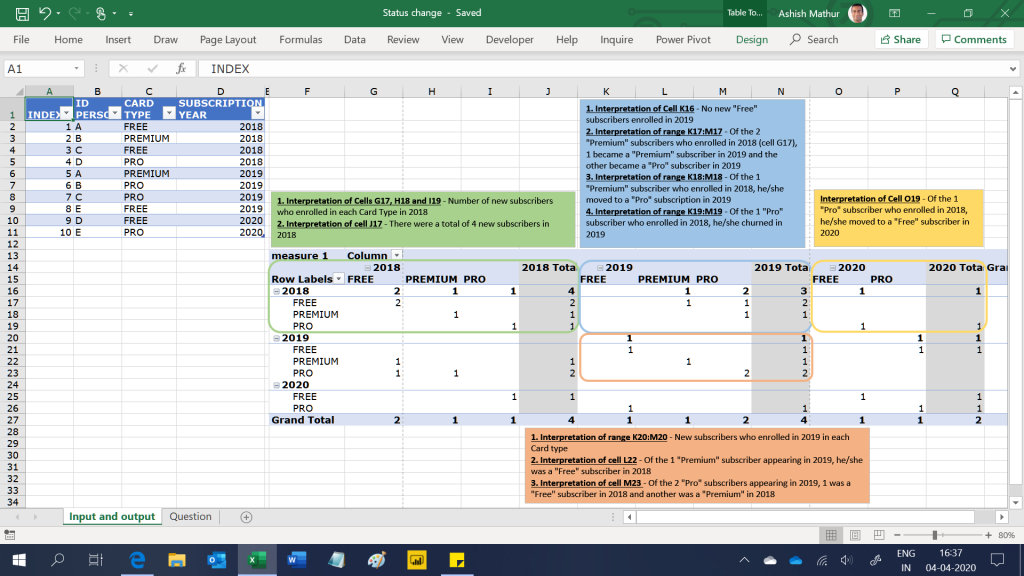I have solved this question using the PowerPivot.  You may download my MS Excel workbook from here.

Here is a sample dataset downloaded from an external application.  As you can observe, there are anomalies in the dataset:1. All the data appears in a single column when Infact it should be segregated into different columns; and
2. There is no separating one record from another.  All we know is that each record ends with a number.  So:
1. Record1 starts at row 2 and ends at row 5
2. Record2 starts at row 6 and ends at row 9
3. Record3 starts at 14 and ends at row 17
3. Not all records have 4 rows.  As an exception, the highlighted range below has 5 rows (row 82 to 86).  The text entry "Part paid" appears in row 85.  In no other record is there the payment status before the number.The objective is to clean the dataset and transform it into a 5 column one as shown below:I have solved this question using the Query Editor (available in both MS Excel and PowerBI Desktop).  You may download my solution workbook from here.

In the value area section of a normal Pivot Table one can only show the result of aggregation functions such as SUM(), COUNT(), AVERAGE() etc.  Even if one drags a text field to the value area section of a Pivot Table, one cannot show those text fields because they automatically get counted.

Consider the following dataset.  The important columns to consider here are COD (Column C), Level (Column E) and Date (column G).For a COD, there can be a number of rows (COD 31512268 has 3 rows).  For this COD, there is just one level (E) for the same date/time.It is also possible that for a particular COD, there can be different Levels (COD 31512259 has 4 rows).  For this COD, there are 2 levels (E and D) for the same data/time.To further complicate the issue, there can be some cases where for the same date/time, a COD may have different levels.  COD 11058698 has 2 different levels (K and M) for the same date/time.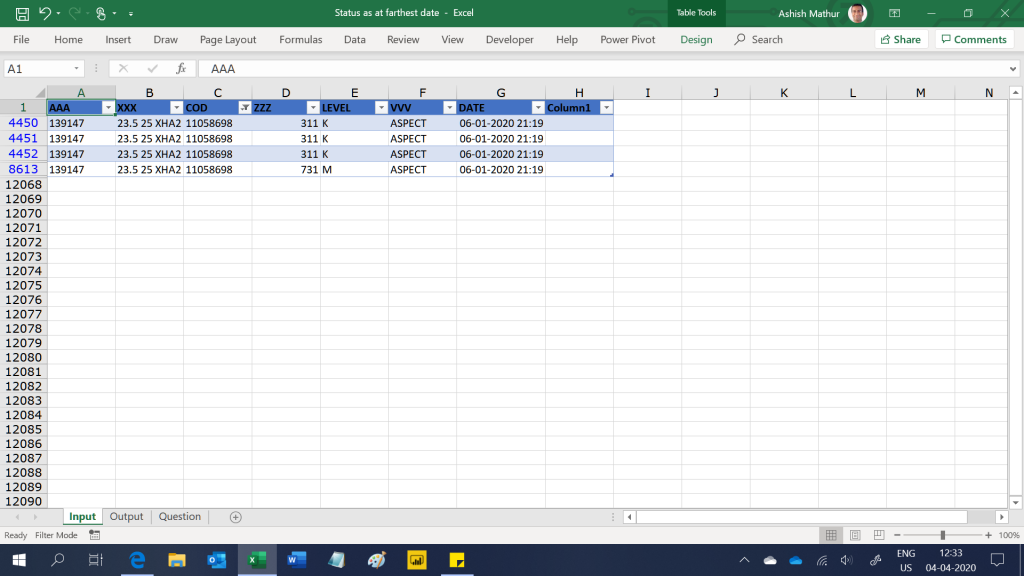The expected result is to show a Pivot Table with COD's in the row labels and the Level(s) as on the farthest date/time of each COD.  If a particular COD has 2 levels as on the farthest date/time, then they should be shown in the value area section of the Pivot Table (separated by commas).  So the expected result should look like this.  Notice that COD 11058698 has 2 levels as on the farthest date/time (K and M) and COD 11058700 has 3 levels as on the farthest date/time (Blank, M and 1M).I have solved this question in MS Excel and PowerBI Desktop with the help of the DAX formulas.  You may download my Excel solution workbook from here and PowerBI Desktop file from here.

Assume a simple 3 column dataset as shown below - the date of each task and the status of that task.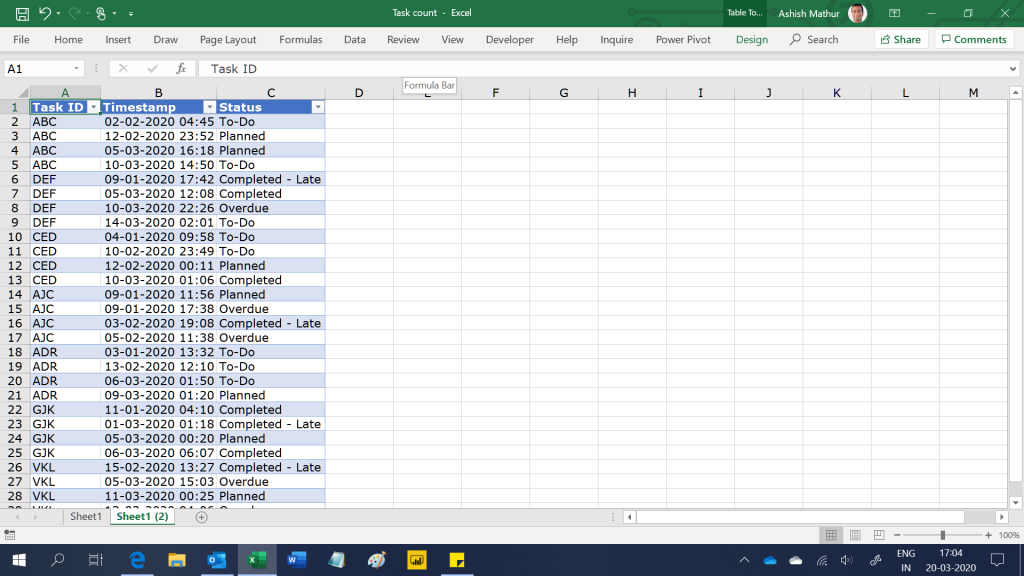The objective is to get the status wise count of tasks by the last time stamp.  So for the Status "To-do", the count should be 2 - Task ABC and DEF.  Only these two tasks on their last time stamp have the status as "To-do".  Tasks CED and ADR should not be counted because their last time stamp had a status other than "To-do".  So the final expected result in MS Excel is: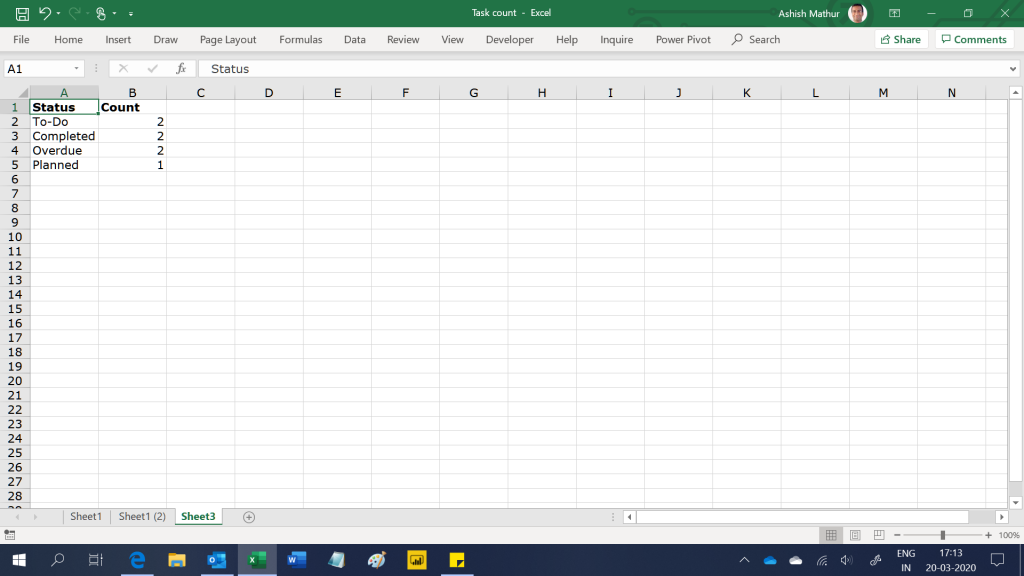Since the original data is being fetched from an external data source, no additional tables or columns can be created from/in the source data table.

The final result in PBI Desktop is thisYou may download my PowerPivot solution workbook from here and PBI Desktop solution file from here.

This post is an extension to the one I posted here - Segment towns according to volume contribution and market share. Here's a simple dataset of Shampoo sales in the state of Rajasthan, India.For a chosen segment, one may want to segment the 4 towns based on the following conditions:Based on the two screenshots shared above, the desired result is shown in the screenshot below:The difference between this solution at the previous one (the link of which I have shared above) is that in this one we want to drag the Classification (range E16:E17) to either the row/column/report filter section of the Pivot Table use it as a slicer.  The current limitation with measures that one writes in PowerPivot's is that measures cannot be used in either row/column/report filter section or as a slicer of/in a Pivot Table.  So in the previous solution, I had written a measure to return the result as Headroom, Stronghold, Emerging or small in only the value area section of the Pivot Table.  One could not drag that measure into the row labels of a Pivot Table.  In this solution, one can drag the Town classification to the row/column/report filter section or even to the slicer (see images below)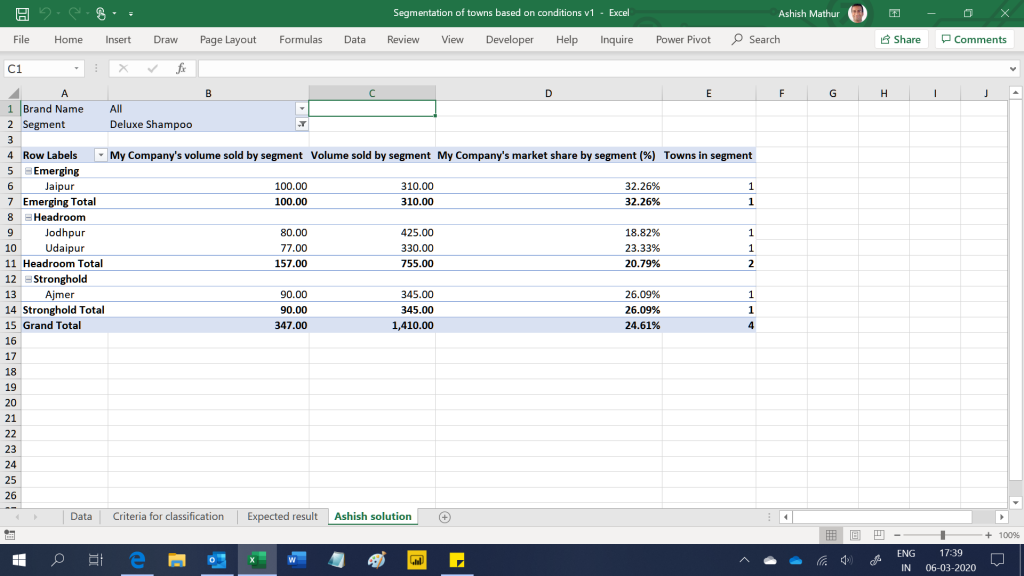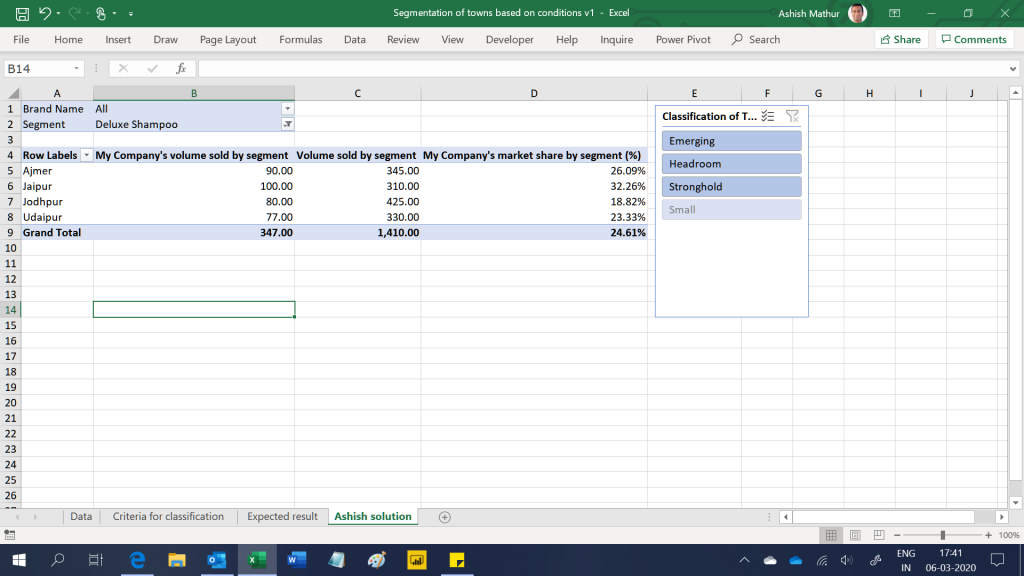Here's a simple dataset of Shampoo sales in the state of Rajasthan, India.For a chosen segment, one may want to segment the 4 towns based on the following conditions:Based on the two screenshots shared above, the desired result is shown in the screenshot below: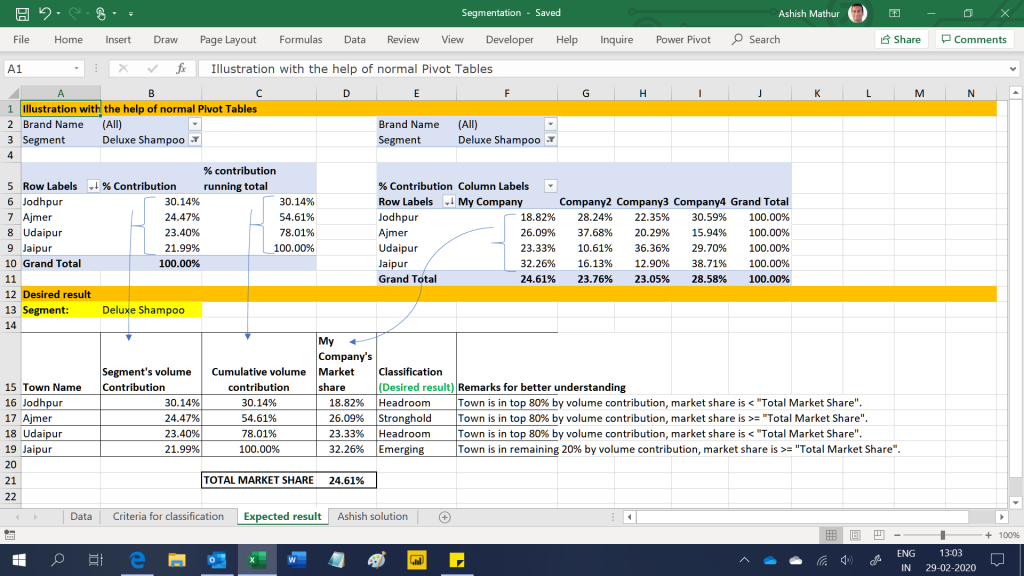The desired result is shown in range E16:E19 and the explanation of the classification is shown in range F16:F19.

The final result obtained by using the PowerPivot is shown in the screenshot below:Many a times data downloaded from Applications/ERP's are not in a filter/Pivot ready format.  In such cases, a lot of time has to first be invested in getting that data in proper order before even beginning to analyse that data.  What makes this situation worse is that data is downloaded every month in that unstructured manner and the effort which was spent on "cleaning up" the data in the previous month has to be replicated this month as well.

Here is one such dataset.  Some easy to spot problems here are:

1. All data is in a single column instead of being in multiple columns
2. Data which should ideally appear in a single row is appearing in multiple rows.  See data in rows 26 to 32.  Information from rows 27 to 32 should appear in row 26
3. Rows 1 to 6 are not required at all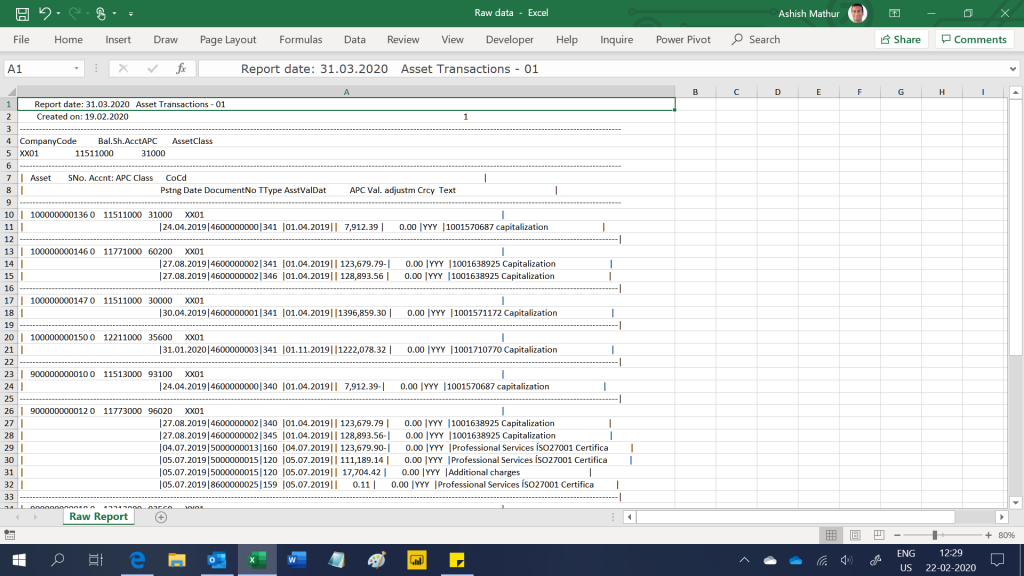Data should appear in the following format to make it "filter/Pivot ready".  Furthermore, when multiple such files (as the one shown above) are placed in a certain folder, one should simply be able to open the output file (shown below) and go to Data > Refresh All.I  have been able to solve this problem using Data > Get & Transform.  Following these steps to get my solution up and working on your system: